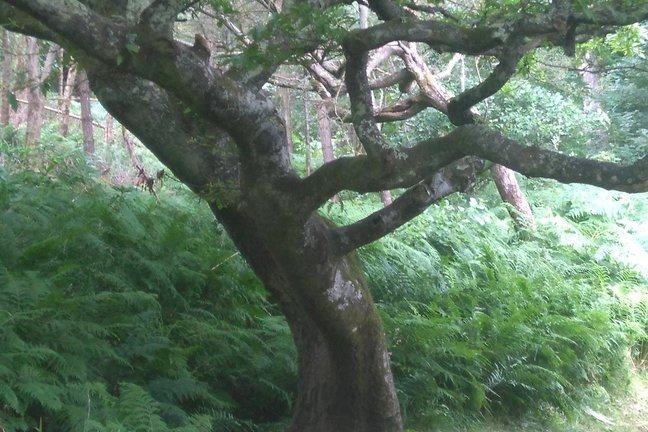## Want to keep learning?

This content is taken from the The University of Glasgow's online course, Functional Programming in Haskell: Supercharge Your Coding. Join the course to learn more.
3.10

## The University of GlasgowA tree

# Grow a Tree

In Computer Science, trees grow upside-down. The unique root
is at the top, and the leaves are at the bottom.

The binary tree data type is often used for storing data in a sorted order, to allow efficient searching — for example, a telephone directory.

We are going to define a Haskell data type for trees, storing integer values.

data Tree = Leaf | Node Int Tree Tree deriving Show


A Tree value might be either a Leaf or a Node. Note that this is a recursive data type, since a Node stores an Int payload and has branches to two subtrees (sometimes called children).

Here is the simplest tree — it’s just a single leaf.

Leaf


Here is a tree with one Node containing value 3, and two leaves.

Node 3 Leaf Leaf


If you type this into ghci, you will see the values returned when you construct these trees, so long as your Tree datatype derives the Show type class.

Look at the type of this value in ghci :

let l = Node 3 Leaf Leaf
:t l


Look at the type of the constructor Node:

:t Node


This is a function: the constructor Node takes three arguments and returns a Tree result.

Now let’s write a function to compute the depth of a Tree — this is the maximum number of branches from the root to any leaf. To write this function, we will do pattern matching on the different kinds of Tree, i.e. Leaf and Node values. Each Leaf is a base case, but for each Node, we need to recursively process the two child trees.

treeDepth :: Tree -> Int
treeDepth Leaf = 0
treeDepth (Node _ leftSubtree rightSubtree) =
1 + max (treeDepth leftSubtree) (treeDepth rightSubtree)


Notice the _ in line 3, which is a ‘don’t care’ value, since we discard the Int payload in each Node.

You can imagine how to write a very similar function that traverses a tree and adds up all the values in its nodes. Have a go at writing this, maybe call it treeSum.

Here is its type:

treeSum :: Tree -> Int


How about a function to check whether a tree is sorted properly? The data structure invariant we want is that, for any Node storing value x, all values in its left subtree are < x, and all values in its right subtree are >= x.

So this function will take in a Tree, a minimum value, a maximum value and it will return a Bool. isSortedTree :: Tree -> Int -> Int -> Bool

A Leaf is automatically sorted, since it does not contain a value. For each Node, we have to check the value in it is between the min and max values, which start off as far apart as possible, then get split into smaller ranges based on the value at the Node.

isSortedTree :: Tree -> Int -> Int -> Bool
isSortedTree Leaf _ _ = True
isSortedTree (Node x leftSubtree rightSubtree) minVal maxVal =
let leftSorted   = isSortedTree leftSubtree minVal x
rightSorted = isSortedTree rightSubtree x maxVal
in x >= minVal && x< maxVal && leftSorted && rightSorted


(assuming you are in right directory) then invoke the function from the ghci prompt:

isSortedTree (Node 2 (Node 1 Leaf Leaf) (Node 3 Leaf Leaf)) minBound maxBound


where minBound is defined as the smallest possible Int value, and maxBound is the largest.

Let’s look at one more function for now. So far, we have studied tree traversal functions, where we go through the tree data structure and do some incremental computation at each node. Now we want to make a tree modification function. This generates a new Tree that is a modified version of the input Tree.

The particular function we are going to define inserts a new maximum value. We go through the input Tree until we find the rightmost node with a Leaf on the right, then we then replace this rightmost Leaf with a new Node containing a new max value (one larger than previous max value).

addNewMax :: Tree -> Tree
-- add a new max element to tree
addNewMax Leaf = Node 0 Leaf Leaf  -- input tree with no nodes
addNewMax (Node x t1 Leaf) = Node x t1 (Node (x+1) Leaf Leaf)  -- this is the rightmost Node
addNewMax (Node x t1 t2) = Node x t1 (addNewMax t2) -- intermediate node, go down right subtree


This addNewMax function takes a Tree input value and returns a Tree output value. You can download this code from addnewmax.hs below, and load it into ghci. Note the function does not do a destructive update — the old input Tree remains unchanged. We have created a new data structure, which has some nodes shared with the original Tree. Search online for purely functional data structures to find out more information about this concept.

Next you need to write some Tree functions for yourself. Download the sortedtree.hs file below and load it into GHCi. Can you write functions to insert a value into a Tree in order, or to convert from a Tree into a list?